# Angular Momentum

Definition: Angular Momentum

The angular momentum, L of a rigid body with moment of inertia I rotating with angular velocity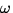is:

 L = I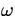. (14)

This is the rotational analogue of linear momentum.
Note: The units of angular momentum are kgm 2/s.

The dynamical torque equation can be written in terms of angular momemtum: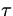= I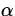= I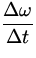=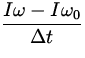=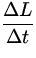. (15)
This is the rotational analogue of Newton's second law:=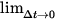.

Idea: Conservation of Angular Momentum

In the absence of external torque (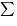= 0) , the angular momentum of a rotating rigid body is conservedL = 0 , or

 Ii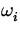= If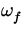. (16)

For systems that consist of many rigid bodies and/or particles, the total angular momentum about any axis is the sum of the individual angular momenta. The conservation of angular moment also applies to such systems. In the absence of external forces acting on the system, the total angular momentum of the system remains constant.

Note:

• Angular momentum and torque are really vector quantities. For two dimensional motion they always point either out of the page (if they are positive) or into the page (if they are negative). Thus we don't need to explicitly consider their vector properties. We need only insure that we have the correct sign.
• Table 8.1 gives the rotational analogues of some linear quantities.
 Linear Stuff Angular Stuff Quantity Units Quantity Units a m/s 2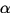rads / s 2 m kg I kgm 2 p = mv kgm/s L = Ikgm 2 /s KEt =mv 2 J KEr =IJ F = ma N= INmNext: Problem Solving Strategies Up: Rotational Equilibrium and Dynamics Previous: Rotational Kinetic Energy